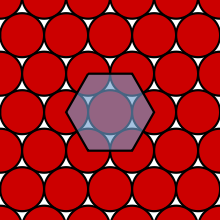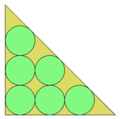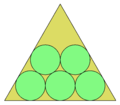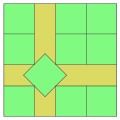# Packing problem

Packing problem

Packing problems are a class of optimization problems in mathematics which involve attempting to pack objects together (often inside a container), as densely as possible. Many of these problems can be related to real life packaging, storage and transportation issues. Each packing problem has a dual covering problem, which asks how many of the same objects are required to completely cover every region of the container, where objects are allowed to overlap.

In a packing problem, you are given:

• 'containers' (usually a single two- or three-dimensional convex region, or an infinite space)
• 'goods' (usually a single type of shape), some or all of which must be packed into this container

Usually the packing must be without overlaps between goods and other goods or the container walls. The aim is to find the configuration with the maximal density. In some variants the overlapping (of goods with each other and/or with the boundary of the container) is allowed but should be minimized.

## Packing infinite space

Many of these problems, when the container size is increased in all directions, become equivalent to the problem of packing objects as densely as possible in infinite Euclidean space. This problem is relevant to a number of scientific disciplines, and has received significant attention. The Kepler conjecture postulated an optimal solution for packing spheres hundreds of years before it was proven correct by Thomas Callister Hales. Many other shapes have received attention, including ellipsoids, Platonic and Archimedean solids including tetrahedra, and unequal-sphere dimers.

### Hexagonal packing of circlesThe hexagonal packing of circles on a 2-dimensional Euclidean plane.

These problems are mathematically distinct from the ideas in the circle packing theorem. The related circle packing problem deals with packing circles, possibly of different sizes, on a surface, for instance the plane or a sphere.

The counterparts of a circle in other dimensions can never be packed with complete efficiency in dimensions larger than one (in a one dimensional universe, the circle analogue is just two points). That is, there will always be unused space if you are only packing circles. The most efficient way of packing circles, hexagonal packing, produces approximately 91% efficiency.

### Sphere packings in higher dimensions

In three dimensions, the face-centered cubic lattice offers the best lattice packing of spheres, and is believed to be the optimal of all packings. The 8-dimensional E8 lattice and 24-dimensional Leech lattice are also believed to be optimal.

### Packings of Platonic solids in three dimensions

Cubes can easily be arranged to fill three-dimensional space completely, the most natural packing being the cubic honeycomb. No other Platonic solid can tile space on its own, but some preliminary results are known. Tetrahedra can achieve a packing of at least 85%. One of the best packings of regular dodecahedra is based on the aforementioned face-centered cubic (FCC) lattice.

Tetrahedra and octahedra together can fill all of space in an arrangement known as the tetrahedral-octahedral honeycomb.

Solid Maximum known packing density Lowest upper bound for lattice packing density
icosahedra 0.836315 0.836357
dodecahedra 0.904002 (5+sqrt(5))/8=0.904508
octahedra 0.947003 18/19 = 0.947368

## Packing in 3-dimensional containers

### Spheres into a Euclidean ball

The problem of finding the smallest ball such that k disjoint open unit balls may be packed inside it has a simple and complete answer in n-dimensional Euclidean space if$k\leq n+1$, and in an infinite dimensional Hilbert space with no restrictions. It is worth describing in detail here, to give a flavor of the general problem. In this case, a configuration of k pairwise tangent unit balls is available. Place the centers at the vertices a1,..,ak of a regular$\scriptstyle(k-1)$ dimensional simplex with edge 2; this is easily realized starting from an orthonormal basis. A small computation shows that the distance of each vertex from the barycenter is$\scriptstyle\sqrt{2\big(1-\frac{1}{k} \big)}$. Moreover, any other point of the space necessarily has a larger distance from at least one of the$k$ vertices. In terms of inclusions of balls, the$k$ open unit balls centered at$a_1,..,a_k$ are included in a ball of radius$r_k:=1+\sqrt{2\big(1-\frac{1}{k}\big)}$, which is minimal for this configuration.

To show that this configuration is optimal, let$x_1,...,x_k$ be the centers of$k$ disjoint open unit balls contained in a ball of radius$r$ centered at a point$x_0$. Consider the map from the finite set$\scriptstyle\{x_1,..x_k\}$ into$\scriptstyle\{a_1,..a_k\}$ taking$x_j$ in the corresponding$a_j$ for each$1\leq j\leq k$. Since for all$1\leq i,$\|a_i-a_j\|=2\leq\|x_i-x_j\|$ this map is 1-Lipschitz and by the Kirszbraun theorem it extends to a 1-Lipschitz map that is globally defined; in particular, there exists a point$a_0$ such that for all$\scriptstyle1\leq j\leq k$ one has$\scriptstyle\|a_0-a_j\|\leq\|x_0-x_j\|$, so that also$r_k\leq1+\|a_0-a_j\|\leq 1+\|x_0-x_j\|\leq r$. This shows that there are$k$ disjoint unit open balls in a ball of radius$r$ if and only if$r\geq r_k$. Notice that in an infinite dimensional Hilbert space this implies that there are infinitely many disjoint open unit balls inside a ball of radius$r$ if and only if$r\geq 1+\sqrt{2}$. For instance, the unit balls centered at$\scriptstyle\sqrt{2}e_j$, where$\scriptstyle\{e_j\}_j$ is an orthonormal basis, are disjoint and included in a ball of radius$1+\sqrt{2}$ centered at the origin. Moreover, for$r<1+\sqrt{2}$, the maximum number of disjoint open unit balls inside a ball of radius r is$\scriptstyle\big\lfloor \frac{2}{2-(r-1)^2}\big\rfloor$.

### Spheres in a cuboid

Determine the number of spherical objects of given diameter d can be packed into a cuboid of size a × b × c.

## Packing in 2-dimensional containers

### Packing circles

#### Circles in circleThe optimal packing of 10 circles in a circle.

Pack n unit circles into the smallest possible circle. This is closely related to spreading points in a unit circle with the objective of finding the greatest minimal separation, dn, between points.

Optimal solutions have been proven for n≤13, and n=19.

#### Circles in squareThe optimal packing of 15 circles in a square.

Pack n unit circles into the smallest possible square. This is closely related to spreading points in a unit square with the objective of finding the greatest minimal separation, dn, between points. To convert between these two formulations of the problem, the square side for unit circles will be L=2+2/dn.

Optimal solutions have been proven for n≤30.

#### Circles in isosceles right triangleThe optimal packing of 6 circles in a right isosceles triangle.

Pack n unit circles into the smallest possible isosceles right triangle. Good estimates are known for n<300.

#### Circles in equilateral triangleThe optimal packing of 5 circles in an equilateral triangle.

Pack n unit circles into the smallest possible equilateral triangle. Optimal solutions are known for n<13, and conjectures are avaiable for n<28.

### Packing squares

#### Squares in squareThe optimal packing of 10 squares in a square.

Pack n unit squares into the smallest possible square.

Optimal solutions have been proven for n=1-10, 14-16, 23-25, 34-36, 62-64, 79-81, 98-100, and any square integer.

The wasted space is asymptotically o(a7/11).

#### Squares in circle

Pack n squares in the smallest possible circle.

Minimum solutions:[citation needed]

1 0.707...
2 1.118...
3 1.288...
4 1.414...
5 1.581...
6 1.688...
7 1.802...
8 1.978...
9 2.077...
10 2.121...
11 2.215...
12 2.236...

### Packing rectangles

#### Identical rectangles in a rectangle

The problem of packing multiple instances of a single rectangle of size (l,w), allowing for 90o rotation, in a bigger rectangle of size (L,W) has some applications such as loading of boxes on pallets and, specifically, woodpulp stowage.

For example, it is possible to pack 147 rectangles of size (137,95) in a rectangle of size (1600,1230).

#### Different rectangles in a rectangle

The problem of packing multiple rectangles of varying widths and heights in an enclosing rectangle of minimum area (but with no boundaries on the enclosing rectangle's width or height) has an important application in combining images into a single larger image. A web page that loads a single larger image often renders faster in the browser than the same page loading multiple small images, due to the overhead involved in requesting each image from the web server.

An example of a fast algorithm that packs rectangles of varying widths and heights into an enclosing rectangle of minimum area is here.

## Related fields

In tiling or tesselation problems, there are to be no gaps, nor overlaps. Many of the puzzles of this type involve packing rectangles or polyominoes into a larger rectangle or other square-like shape.

There are significant theorems on tiling rectangles (and cuboids) in rectangles (cuboids) with no gaps or overlaps:

Klarner's theorem: An a × b rectangle can be packed with 1 × n strips iff n | a or n | b.
de Bruijn's theorem: A box can be packed with a harmonic brick a × a b × a b c if the box has dimensions a p × a b q × a b c r for some natural numbers p, q, r (i.e., the box is a multiple of the brick.)

The study of polyomino tilings largely concerns two classes of problems: to tile a rectangle with congruent tiles, and to pack one of each n-omino into a rectangle.

A classic puzzle of the second kind is to arrange all twelve pentominoes into rectangles sized 3×20, 4×15, 5×12 or 6×10.

## Packing of irregular objects

Packing of irregular objects is a problem not lending itself well to closed form solutions; however, the applicability to practical environmental science is quite important. For example, irregularly shaped soil particles pack differently as the sizes and shapes vary, leading to important outcomes for plant species to adapt root formations and to allow water movement in the soil.

Wikimedia Foundation. 2010.

### Look at other dictionaries:

• Bin packing problem — In computational complexity theory, the bin packing problem is a combinatorial NP hard problem. In it, objects of different volumes must be packed into a finite number of bins of capacity V in a way that minimizes the number of bins used.There… …   Wikipedia

• Bin-Packing-Problem — Das Behälterproblem oder auch Bin Packing ist ein kombinatorisches Optimierungsproblem, das auf folgender Fragestellung basiert: Gegeben: Eine Anzahl von „Behältern“ (englisch bin) der Größe und eine Anzahl „Objekte“ mit den Größen …   Deutsch Wikipedia

• Bin Packing Problem — Das Behälterproblem oder auch Bin Packing ist ein kombinatorisches Optimierungsproblem, das auf folgender Fragestellung basiert: Gegeben: Eine Anzahl von „Behältern“ (englisch bin) der Größe und eine Anzahl „Objekte“ mit den Größen …   Deutsch Wikipedia

• Packing — may refer to:In Mechanical engineering: * Packing, also known as an O ring or other type of Seal (mechanical), a term for a sealing material * Packing gland is a mechanical term for the groove in which a packing sits.In Mathematics: * Sphere… …   Wikipedia

• Bin packing problem — Problème de bin packing Le problème de bin packing relève de la recherche opérationnelle et de l optimisation combinatoire. Il s agit de trouver le rangement le plus économique possible pour un ensemble d articles dans des boîtes. Le problème… …   Wikipédia en Français

• Problem of Apollonius — In Euclidean plane geometry, Apollonius problem is to construct circles that are tangent to three given circles in a plane (Figure 1); two circles are tangent if they touch at a single point. Apollonius of Perga (ca. 262 BC ndash; ca. 190 BC)… …   Wikipedia

• packing — /pak ing/, n. 1. the act or work of a person or thing that packs. 2. the preparation and packaging of foodstuffs, esp. to be sold at wholesale. 3. the way in which something is packed. 4. an act or instance of transporting supplies, goods, etc.,… …   Universalium

• Set packing — is a classical NP complete problem in computational complexity theory and combinatorics, and was one of Karp s 21 NP complete problems. Suppose we have a finite set S and a list of subsets of S. Then, the set packing problem asks if some k… …   Wikipedia

• Cutting stock problem — The cutting stock problem is an optimization problem, or more specifically, an integer linear programming problem. It arises from many applications in industry. Imagine that you work in a paper mill and you have a number of rolls of paper of… …   Wikipedia

• Circle packing theorem — Example of the circle packing theorem on K5, the complete graph on five vertices, minus one edge. The circle packing theorem (also known as the Koebe–Andreev–Thurston theorem) describes the possible tangency relations between circles in the plane …   Wikipedia